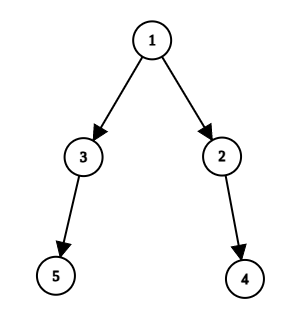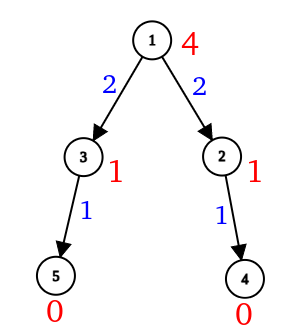Can anyone help me code solution for this question?

My Approach (Based on the fact that this problem is placed under the Trees section. Hope it helps).

• Construct a Tree using the given data.

For instance,

``````5
1 1 2 3

// Parent = 1
// Parent = 1
// Parent = 2
// Parent = 3
``````

This input represents the following tree (rooted at 1).Now, run a DFS, where at each node we perform the following. Each node returns the value `(number_of_subOrdinates + 1)`:

• If the current node is a leaf node (has no children), the number of sub-ordinates for this node is 0. So, a leaf node returns 1 to its parent.
• Else, the number of subordinates for the current node is given by the sum of sub-ordinate values returned by its children.Now, the above image represents the same.

• Values marked in Red represent the number of subordinates of that node.
• Values marked in Blue represent the value returned by a node to its parent.

We can store all these values in an Array while performing DFS.

Finally, we iterate over the array and output these values.

Thanks guys